# HSPT Quantitative : How to work with number series

## Example Questions

← Previous 1 3 4 5 6 7 8 9 20 21

### Example Question #1 : Number Series*

What number should come next in this series?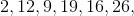__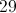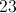Explanation:

The pattern is add, subtract. The last operation is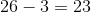.

### Example Question #1 : How To Work With Number Series

What number should come next in this series?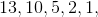__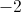Explanation:

The pattern is subtract, divide by. The last operation is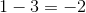.

### Example Question #1 : Number Series*

What number should come next in this series?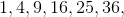__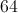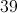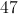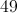Explanation:

The pattern in this series is perfect squares: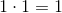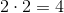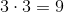...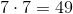### Example Question #2 : How To Work With Number Series

What number should come next in this series?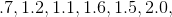__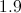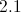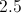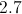Explanation:

The pattern is add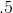, then subtract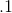.

The last operation is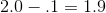.

### Example Question #2 : How To Work With Number Series

What number should come next in this series?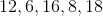, __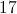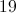Explanation:

The pattern is divide by, then add. The last operation is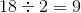.

### Example Question #5 : Number Series*

What number should come next in this series?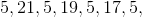__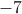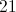Explanation:

The general pattern for this one is subtracteach time, but ais placed between each element. Ignoring the's, the last operation is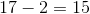.

### Example Question #1 : Number Series*

What number should come next in this series?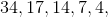__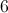Explanation:

The pattern is divide by, then subtract. The last operation is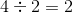.

### Example Question #6 : Number Series*

What number should fill in the blank in this series?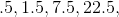__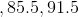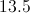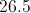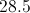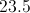Explanation:

The pattern is multiply by, then add. The operation before the blank is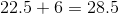.

### Example Question #2 : Number Series*

What number should come next in this series?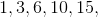__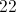Explanation:

Each element in the series adds a number that is one more than the last: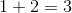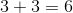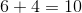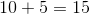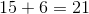### Example Question #2 : Number Series*

What number should come next in this series?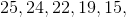__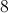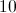Explanation:

In this series, a number is subtracted each time. That number always increases by: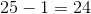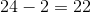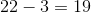...

The last operation is: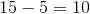← Previous 1 3 4 5 6 7 8 9 20 21